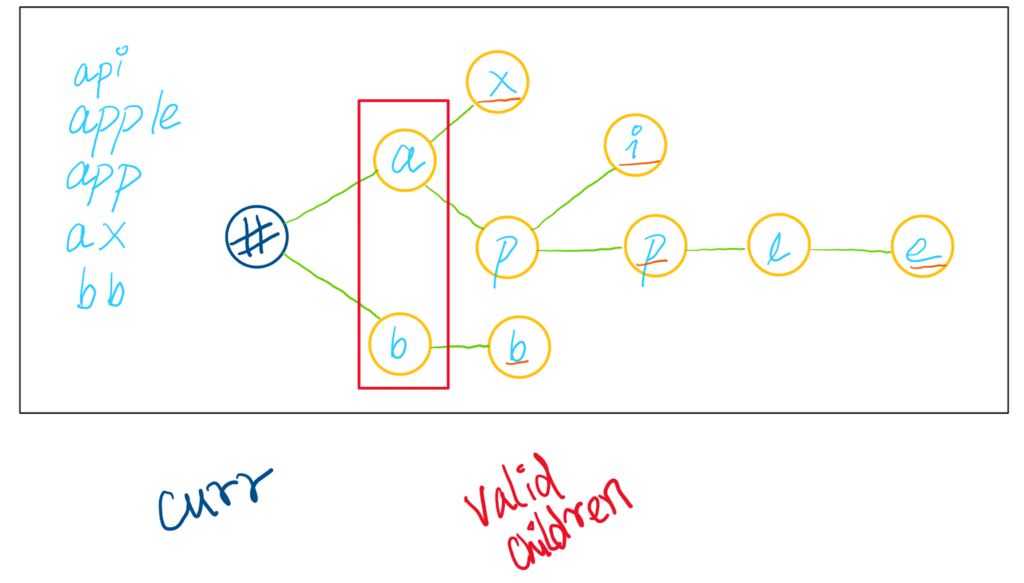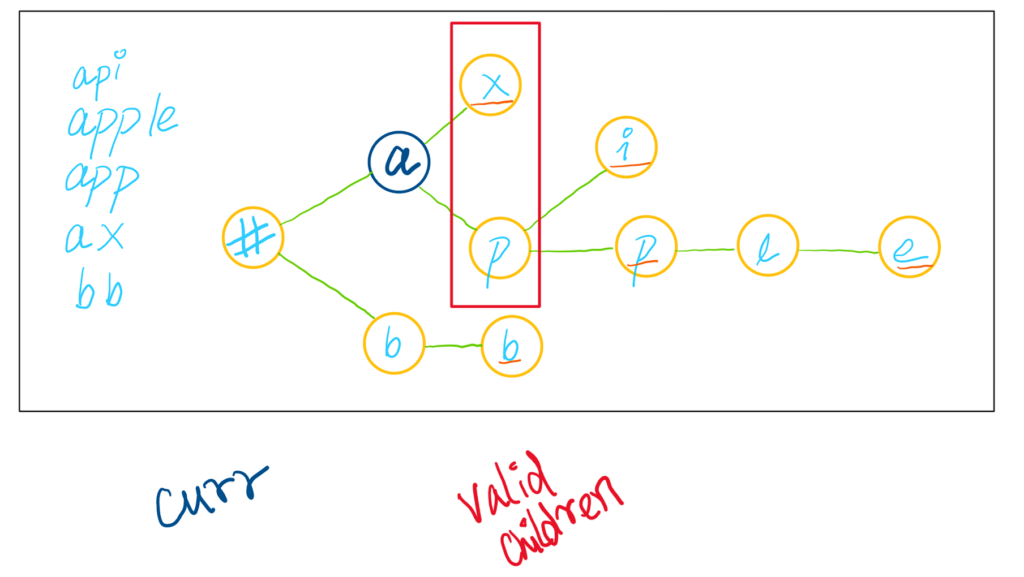# Word Search II | LeetCode | Solution Explained

Word Search II is an amazing problem, building on top of two other problems: Word Search I & Implement Prefix Tree. In this writeup, I explain the solution in detail with Python3 code. The first thing we discuss is the brute force solution and then we realize the use of prefix trees or tries. Only by combining both the ideas we are able to get the solution the sweet-sweet-green accepted. Problem Link.

# Brute force Solution (Time Limit Error)

This code is modified from my previous post: Word Search | LeetCode | Solution Explained. In that solution I also mentioned the time & space complexities as:

• `Time: O(M*N*3^L)` where M and N are the dimensions of the board and L is the length of the input word
• `Space: O(L)` for the recursion call stack height

# Modifying Word Search I Solution

For the question Word Search II, we have to iterate over all the words as well, meaning the time complexity is actually `O(M*N*3^L * W)` where W is the number of words - we have this `W` factor additional. The space complexity is the same. Note that the `ans` list that we store looks like `O(W)` complexity, but its a requirement and not additional space. So space complexity remains the same - the height of the stack.

`class Solution:    def findWords(self, board: List[List[str]], words: List[str]) -> List[str]:        def search(x, y, i, w):            if i == len(w): return True            if not (0 <= x < m and 0 <= y < n): return False            if board[x][y] != w[i]: return False            if board[x][y] == ' ': return False            board[x][y] = ' '                        temp = search(x+1, y, i+1, w) or search(x-1, y, i+1, w) or search(x, y+1, i+1, w) or search(x, y-1, i+1, w)            board[x][y] = w[i]            return temp                m, n = len(board), len(board)        ans = []        for x in range(m):            for y in range(n):                for w in words:                    if board[x][y] == w:                        if search(x, y, 0, w):                             ans.append(w)                words = [w for w in words if w not in ans]                return ans`

# Solution Logic (Accepted)

## Observations & Trie

The core of the solution is based upon the realization that the words themselves are … similar. Take a look at the following test case: `[aaab, aaac, aaaxd]`. What do you note here?

All of these share a common prefix. And what is a data structure that can deal with prefixes efficiently? Trie aka Prefix Tree! As we saw before, the trie looks something of the sort shown below, with different examples.

An example of trie data structure storing prefixes efficiently

If you are unclear, refer Implement Trie (Prefix Tree) | LeetCode | Solution Explained. I have explained the Trie data structure in detail.

## Extending Word Search I to Word Search II

We then apply the concepts seen from the problem Word Search | LeetCode | Solution Explained. The parallels between them can be explained as such:

The `search` function for this problem will be modified a bit, since we also want to keep a track of the words themselves. This is why we see another variable path, which starts off as an empty string and we keep adding characters to keep, so that we know what the word is, when we are saving it. The following is the code for it.

# Word Search II Accepted Code

The code for Node and Trie is taken from Implement Trie (Prefix Tree) | LeetCode | Solution Explained.

`class Node:    def __init__(self, c, end=False):        self.c = c         self.child = {}        self.end = end        class Trie:    def __init__(self):        self.root = Node('#')def insert(self, word: str) -> None:        curr = self.root        for c in word:            if c not in curr.child:                 curr.child[c] = Node(c)            curr = curr.child[c]        curr.end = Trueclass Solution:    def findWords(self, board: List[List[str]], words: List[str]) -> List[str]:        def search(x, y, curr, path):           # if not in the grid, we don't care            if not (0 <= x < m and 0 <= y < n): return                        c = board[x][y]                        # if character is not in the data structure, we have no use for it            # explained in detail below            if c not in curr.child: return             if curr.child[c].end:                 ans.add(path+c) # if that character marks the end                # we don't return here, since there can be a case like:                # app & apple.                # if you return at app, apple would never get considered                        board[x][y] = ' '            search(x+1, y, curr.child[c], path+c) # D            search(x-1, y, curr.child[c], path+c) # U            search(x, y+1, curr.child[c], path+c) # R             search(x, y-1, curr.child[c], path+c) # L            board[x][y] = c                trie = Trie()        for word in words: trie.insert(word)                curr = trie.root        m, n = len(board), len(board)        ans = set() # because there may be duplicate cases, one word can be found multiple times in the grid        for x in range(m):            for y in range(n):                search(x, y, curr, "")               return list(ans)`

The line `if c not in curr.child: return` is a densely packed line. What it means is that we can not go out of the trie or prefix tree data structure. By restricting our character space, we are able to more efficiently traverse the grid. Take for example the case below. The `curr` is the `#` character. Its possible children are ONLY `a` and `b`. If the current `c = board[x][y]` is NOT in the `valid_children` list, then we can return right away.Representation of Word Search II Trie or Prefix Tree, in the first iteration

If however, `c = board[x][y]` is present inside the list, we can move forward like so:Representation of Word Search II Trie or Prefix Tree, in the next iteration

And this is it! Thanks for reading 😀

Originally published at https://chaudhary1337.com on October 9, 2021.

--

--

--

## More from Tanishq Chaudhary

Trying out some stuff.

Love podcasts or audiobooks? Learn on the go with our new app.## Tanishq Chaudhary

Trying out some stuff.

## Outreachy: Halfway through the journey